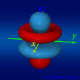# 什么是生成函数？

我们年级有许多漂亮的MM。一班有7个左右吧，二班大概有4个，三班最多，16个，四班最可怜，一个漂亮的MM都没有，五班据说有1个。如果用一个函数“f(班级)=漂亮MM的个数”，那么我们可以把上述信息表示成：f(1)=7,f(2)=4,f(3)=16,f(4)=0,f(5)=1,等等。
生成函数（也有叫做“母函数”的，但是我觉得母函数不太好听）是说，构造这么一个多项式函数g(x)，使得x的n次方系数为f(n)。于是，上面的f函数的生成函数g(x)=7x+4x^2+16x^3+x^5+…。这就是传说中的生成函数了。关键是，这个有什么用呢？一会儿要慢慢说。我敢打赌这绝对会是我写过的最长的一篇文章。

生成函数最绝妙的是，某些生成函数可以化简为一个很简单的函数。也就是说，不一定每个生成函数都是用一长串多项式来表示的。比如，这个函数f(n)=1 （n当然是属于自然数的），它的生成函数就应该是g(x)=1+x+x^2+x^3+x^4+…（每一项都是一，即使n=0时也有x^0系数为1，所以有常数项）。再仔细一看，这就是一个有无穷多项的等比数列求和嘛。如果-1<x<1，那么g(x)就等于1/(1-x)了。在研究生成函数时，我们都假设级数收敛，因为生成函数的x没有实际意义，我们可以任意取值。于是，我们就说，f(n)=1的生成函数是g(x)=1/(1-x)。

我们举一个例子说明，一些具有实际意义的组合问题也可以用像这样简单的一个函数全部表示出来。
考虑这个问题：从二班选n个MM出来有多少种选法。学过简单的排列与组合的同学都知道，答案就是C(4,n)。也就是说。从n=0开始，问题的答案分别是1,4,6,4,1,0,0,0,…（从4个MM中选出4个以上的人来方案数当然为0喽）。那么它的生成函数g(x)就应该是g(x)=1+4x+6x^2+4x^3+x^4。这不就是……二项式展开吗？于是，g(x)=(1+x)^4。
你或许应该知道，(1+x)^k=C(k,0)x^0+C(k,1)x^1+…+C(k,k)x^k；但你或许不知道，即使k为负数和小数的时候，也有类似的结论：(1+x)^k=C(k,0)x^0+C(k,1)x^1+…+C(k,k)x^k+C(k,k+1)x^(k+1)+C(k,k+2)x^(k+2)+…（一直加到无穷；式子看着很别扭，自己写到草稿纸上吧，毕竟这里输入数学式子很麻烦）。其中，广义的组合数C(k,i)就等于k(k-1)(k-2)…(k-i+1)/i!，比如C(4,6)=4*3*2*1*0*(-1)/6!=0，再比如C(-1.4,2)=(-1.4)*(-2.4)/2!=1.68。后面这个就叫做牛顿二项式定理。当k为整数时，所有i>k时的C(k,i)中分子都要“越过”0这一项，因此后面C(k,k+1),C(k,k+2)之类的都为0了，与我们的经典二项式定理结论相同；不同的是，牛顿二项式定理中的指数k可以是任意实数。

我们再举一个例子说明一些更复杂的生成函数。n=x1+x2+x3+…+xk有多少个非负整数解？这道题是学排列与组合的经典例题了。把每组解的每个数都加1，就变成n+k=x1+x2+x3+…+xk的正整数解的个数了。教材上或许会出现这么一个难听的名字叫“隔板法”：把n+k个东西排成一排，在n+k-1个空格中插入k-1个“隔板”。答案我们总是知道的，就是C(n+k-1,k-1)。它就等于C(n+k-1,n)。它关于n的生成函数是g(x)=1/(1-x)^k。这个生成函数是怎么来的呢？其实，它就是(1-x)的-k次方。把(1-x)^(-k)按照刚才的牛顿二项式展开，我们就得到了x^n的系数恰好是C(n+k-1,n)，因为C(-k,n)*(-x)^n=[(-1)^n*C(n+k-1,n)]*[(-1)^n*x^n]=C(n+k-1,n)x^n。这里看晕了不要紧，后文有另一种方法可以推导出一模一样的公式。事实上，我们有一个纯组合数学的更简单的解释方法。因为我们刚才的几何级数1+x+x^2+x^3+x^4+…=1/(1-x)，那么(1+x+x^2+x^3+x^4+…)^k就等于1/(1-x)^k。仔细想想k个(1+x+x^2+x^3+x^4+…)相乘是什么意思。(1+x+x^2+x^3+x^4+…)^k的展开式中，n次项的系数就是我们的答案，因为它的这个系数是由原式完全展开后k个指数加起来恰好等于n的项合并起来得到的。

现在我们引用《组合数学》上暴经典的一个例题。很多书上都会有这类题。
我们要从苹果、香蕉、橘子和梨中拿一些水果出来，要求苹果只能拿偶数个，香蕉的个数要是5的倍数，橘子最多拿4个，梨要么不拿，要么只能拿一个。问按这样的要求拿n个水果的方案数。
结合刚才的k个(1+x+x^2+x^3+x^4+…)相乘，我们也可以算出这个问题的生成函数。

g(x)=(1+x^2+x^4+…)(1+x^5+x^10+..)(1+x+x^2+x^3+x^4)(1+x)
=[1/(1-x^2)]*[1/(1-x^5)]*[(1-x^5)/(1-x)]*(1+x) （前两个分别是公比为2和5的几何级数，
第三个嘛，(1+x+x^2+x^3+x^4)*(1-x)不就是1-x^5了吗）
=1/(1-x)^2   （约分，把一大半都约掉了）
=(1-x)^(-2)=C(1,0)+C(2,1)x+C(3,2)x^2+C(4,3)x^3…   （参见刚才对1/(1-x)^k的展开）
=1+2x+3x^2+4x^3+5x^4+….

于是，拿n个水果有n+1种方法。我们利用生成函数，完全使用代数手段得到了答案！
如果你对1/(1-x)^k的展开还不熟悉，我们这里再介绍一个更加简单和精妙的手段来解释1/(1-x)^2=1+2x+3x^2+4x^3+5x^4+….。
1/(1-x)=1+x+x^2+x^3+x^4+…是前面说过的。我们对这个式子等号两边同时求导数。于是，1/(1-x)^2=1+2x+3x^2+4x^3+5x^4+….。一步就得到了我们所需要的东西！不断地再求导数，我们同样可以得到刚才用复杂的牛顿二项式定理得到的那个结论（自己试试吧）。生成函数还有很多其它的处理手段，比如等式两边同时乘以、除以常数（相当于等式右边每一项乘以、除以常数），等式两边同时乘以、除以一个x（相当于等式右边的系数“移一位”），以及求微分积分等。神奇的生成函数啊。
我们用两种方法得到了这样一个公式：1/(1-x)^n=1+C(n,1)x^1+C(n+1,2)x^2+C(n+2,3)x^3+…+C(n+k-1,k)x^k+…。这个公式非常有用，是把一个生成函数还原为数列的武器。而且还是核武器。

接下来我们要演示如何使用生成函数求出Fibonacci数列的通项公式。
Fibonacci数列是这样一个递推数列：f(n)=f(n-1)+f(n-2)。现在我们需要求出它的生成函数g(x)。g(x)应该是一个这样的函数：
g(x)=x+x^2+2x^3+3x^4+5x^5+8x^6+13x^7+…
等式两边同时乘以x，我们得到：
x*g(x)=x^2+x^3+2x^4+3x^5+5x^6+8x^7+…
就像我们前面说过的一样，这相当于等式右边的所有系数向右移动了一位。
现在我们把前面的式子和后面的式子相加，我们得到：
g(x)+x*g(x)=x+2x^2+3x^3+5x^4+8x^5+…
把这最后一个式子和第一个式子好好对比一下。如果第一个式子的系数往左边移动一位，然后把多余的“1”去掉，就变成了最后一个式子了。由于递推函数的性质，我们神奇地得到了：g(x)+x*g(x)=g(x)/x-1。也就是说，g(x)*x^2+g(x)*x-g(x)=-x。把左边的g(x)提出来，我们有：g(x)(x^2+x-1)=-x。于是，我们得

## 36 条评论

•dd

最后两行纯属多余……只要看到第一句话就会想到作者的……

回复：保持一种写作风格不但可以当作无形的签名，还可以减少转载（转载一个到处提到MM的OI文章还是要好好考虑一下影响的）

•dd

如果不是我了解你对设计模式一窍不通的话，我会把这篇文章当成你的大作的（无形的签名……）：http://www.pconline.com.cn/pcjob/process/other/others/0412/512340.html
不过那篇文章可是转载无数……

回复：搞计算机的都喜欢MM？

•刺猬

难道不是所有人都喜欢MM吗？
搞计算机的也是人好伐。

•huyichen

我看孙贺的书上有那道提且要求用母函数，不过还是多谢回复，邮票设计问题是一道未解的数论题的通俗描述，这可是一道难题呀

回复：欢迎大家提出自己的想法

•huyichen

不知大牛对DP天牛题的第二题怎么想？

回复：
1.题目太长了，我没看；
2.我还不算是牛人。

•TIME

g(x)=(1+x+x^2+x^3+…)(1+x^2+x^4+…)(1+x^5+x^10+..)=1/[(1-x)(1-x^2)(1-x^5)]。现在，我们需要把它变成通项公式。我们的步骤同刚才的步骤完全相同。我们把(1-x)(1-x^2)(1-x^5)展开，得到1-x-x^2+x^3-x^5+x^6+x^7-x^8。我们求出-1+x+x^2-x^3+x^5-x^6-x^7+x^8=0的解，得到了以下8个解：-1,1,1,1,-(-1)^(1/5),(-1)^(2/5),-(-1)^(3/5),(-1)^(4/5)。这个不是我解出来的，我还是用的Mathematica 5.0。不是我不想解，而是我根本不会解这个8次方程。这也是为什么信息学会涉及这些东西的原
(1+0x^1+0x^2+0x^3+……)/(1-x-x^2+x^3-x^5+x^6+x^7-x^8)
可以?

回复：对不起，我不明白你的意思

•lina

Thanks~[smile]

•cgy4ever

太强大了!

•hplonline

请问地幔是自动的吗？每次从引用点进来都看到这样格式的东西？

•AKIRA

如果我本科的时候就知道这个网站的话就不会没事就在宿舍睡大觉了……相见恨晚啊-3-

•trif

那个8次方程怎么可能不会解？不要把它展开来不就行了么……

•云中子

不是据传母函数都是用来正组合恒等式的么？

•cofidos

函数吓死我了

•x

“我们把(1-x)(1-x^2)(1-x^5)展开，得到1-x-x^2+x^3-x^5+x^6+x^7-x^8。我们求出-1+x+x^2-x^3+x^5-x^6-x^7+x^8=0的解，得到了以下8个解：-1,1,1,1,-(-1)^(1/5),(-1)^(2/5),-(-1)^(3/5),(-1)^(4/5)。这个不是我解出来的，我还是用的Mathematica 5.0。不是我不想解，而是我根本不会解这个8次方程。”

暈倒，不要展開啊！
(1-x) -> 1
(1-x^2) -> 1,-1
(1-x^5) -> 1,1^(1/5),1^(2/5),1^(3/5),1^(4/5)
M67大牛你腫麼了。。。

•minglingmaster

(1+x)^k=C(k,0)x^0+C(k,1)x^1+…+C(k,k)x^k+C(k,k+1)x^(k+1)+C(k,k+2)x^(k+2)+…

这公式有错吧，k如果不为整数，就不能存在C(k,k)吧

•abyssfish

广义的二项式定理

•ww3113306

牛顿二项式定理……

•Ric_shooter

怎么缺了！

•Wuduan

文章为什么显示不全呢？

•12002

显示不全！！！

•亿万先生注册送58元

自己要先看得起自己，别人才会看得起你。

•tan45

(1+x)^k=C(k,0)x^0+C(k,1)x^1+…+C(k,k)x^k+C(k,k+1)x^(k+1)+C(k,k+2)x^(k+2)+…
应该可以把C(k,k+1)及以后的项给删了吧

•CHHC丶_

可以，但没有必要（